Journal of Vibroengineering

Published: 09 February 2023

# An improved deconvolution beamforming algorithm for acoustic imaging of low signal-to-noise ratio sound sources in reverberant field

Wenyong Guo1
Hantao Chen2
Jing Xia3
Xiaofeng Li4
Chenghao Cao5
1, 2, 4, 5College of Power Engineering, Naval University of Engineering, Wuhan, China
3Military Representative Bureau of the Naval Armament Department in Guangzhou, Guangzhou, China
Corresponding Author:
Xiaofeng Li
Views 42

#### Abstract

Most of the existing acoustic imaging studies in reverberant field ignore the influence of signal-to-noise ratio. As a result, commonly used beamforming algorithms in reverberant backgrounds have poor imaging accuracy for low signal-to-noise ratio sound sources. In response to that problem, an improved adaptive beamforming algorithm called SC-DAMAS is put forward in this paper. The algorithm replaces the free-field Green's function with the impulse response function, making the algorithm more suitable for acoustic imaging of low signal-to-noise ratio in a reverberant environment. Besides, the comparative simulation results with the conventional beamforming method and orthogonal matching pursuit algorithm-based DAMAS, as well as sound source acoustic imaging experiments are carried out to analyze its effectiveness. It is indicated that, in the reverberation field, the SC-DAMAS has no obvious sidelobes and achieves higher positioning accuracy for acoustic imaging of low signal-to-noise ratio sound source than the abovementioned counterparts, and its imaging test result is consistent with the actual situation, which verifies the effectiveness of the algorithm.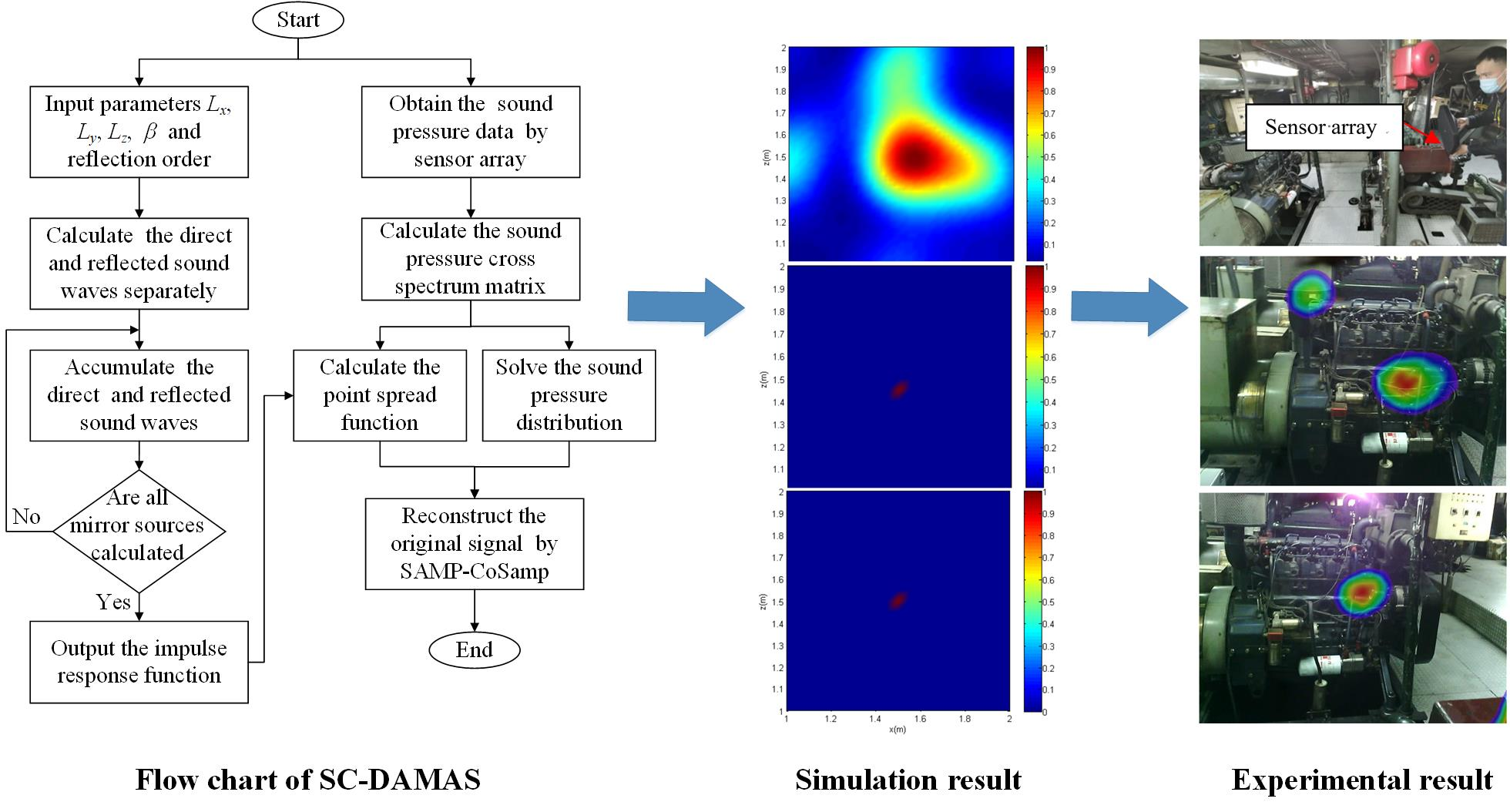#### Highlights

• By introducing the free-field Green's function, an improved adaptive beamforming algorithm, called SC-DAMAS, is put forward in this paper for acoustic imaging of low signal-to-noise ratio in a reverberant environment.
• Comparative simulations among the SC-DAMAS and other classical algorithms in different signal-to-noise ratio scenarios indicate the proposed algorithm has no obvious sidelobes and achieves higher positioning accuracy.
• Sound source acoustic imaging experiments of SC-DAMAS in a real ship engine cabin further show that its imaging result is consistent with the actual situation, which verifies the effectiveness of the algorithm.

## 1. Introduction

A reverberant sound field is formed when sound waves propagate in a closed indoor environment, as they will continuously be reflected and absorbed while encountering walls or other obstacles. In reverberant field, in addition to the direct sound wave from the sound source, the reflected sound after reflections of the original sound wave and external noise is also collected by the sensor array. Therefore, sound source localisation in a reverberant environment is far more complicated than that in the free field, which brings a challenge to the sound source localisation work .

In general, signal processing and acoustic imaging are two main parts of sound sources localisation in a reverberation field. For the signal processing part, compressive sensing (CS) is a widely adopted technology. In the CS technique, signals are acquired by sub-Nyquist rates of traditional signal processing . It gets rid of the limitation that the sampling frequency needs to be at least twice the highest frequency of the original signal to accurately recover the information in the signal . For instance, based on sparse recovery in CS, a multi-channel deconvolution algorithm was proposed to enhance the source signal by Ping et al. . Besides, on the basis of sparse representation, Wang et al.  combined the variational Bayesian expectation-maximization method to solve the equation and studied the sound source localisation in the reverberant environment. Thanks to the previous valuable investigations, more CS reconstruction algorithms have been developed for acoustic beamforming, like Orthogonal Matching Pursuit , Regularized OMP , Compressive Sampling Matching Pursuit (CoSaMP) , Variable step-size Gradient Matching Pursuit , Stochastic Gradient Matching Pursuit  and sparsity adaptive matching pursuit (SAMP) . Among them, the SAMP algorithm is adaptive to its sparsity value but has unstable reconstruction accuracy and sensitive running speed to the iteration step size. More specifically, too small a step size can lead to insufficient sparsity estimation, which increases the number of algorithm iterations and thereby reduces the algorithm efficiency. On the other hand, sparse overestimation problem is generated with too large a step size, and further negatively affects the reconstruction accuracy of the signal. As for the CoSaMP algorithm, it owns a speed advantage with predetermined sparsity. Therefore, it is inspired that hybrid algorithm combining SAMP and CoSaMP can maintain the speed advantage and adaptive searching ability for sparsity.

As for specific acoustic imaging in a reverberation field, it generally involves the beamforming solution and interference suppression. For instance, combined with the array signal processing technology, Peled et al.  studied the reverberation suppression algorithm in the circular sensor array, which decreases the negative effects of the reflected sound waves. Aiming at acoustic imaging after long reverberation time, a general cross-correlation classification algorithm was proposed by Sun et al.  to improve robustness to reverberation environmental changes. Meanwhile, based on the principle of matrix low-rank characteristics, Xu et al.  reported an improved method, and based on dithering technology, Hao et al.  proposed a GCC-IWF algorithm for underwater reverberation environment. All of them contributed to improving the accuracy of acoustic imaging in a reverberant field. For the interference suppression, Nahma et al.  proposed a robust beamforming algorithm based on the room impulse response, which improved the algorithm's robustness in different reverberant environments. Rajan et al.  used the wavelet denoising method for time delay estimation, which effectively reduced the influence of underwater reverberation on sound source localisation. Jiang et al.  proposed a new algorithm combining deep fusion and Convolutional Neural Network in response to the problem of inaccurate sound source localisation in a reverberant environment. Combining double-wide matching pursuit method, Fang et al.  proposed a multi-sound source localisation counting technique. The sound source location accuracy and absolute error analysis results show that this methodology has better accuracy in the conditions of strong reverberation and multiple sound sources. Kilis et al.  proposed a new speech de-reverberation algorithm and carried out experimental verification in the actual reverberation environment. Fischer et al.  modified the cross-correlation matrix of the array sound pressure signal to effectively improve the beamforming accuracy in the reverberant environment. Based on the statistical characteristics of binaural signals and the difference in amplitude spectrum, Ghamdan et al.  proposed a new algorithm. This algorithm can be applied to different reverberation rooms and weaken the dependence on relevant environmental parameters.

In the real environment, the actual ship cabin generally belongs to a narrow and closed space with unavoidable indoor reverberation, which further aggravates the difficulty of locating the target sound source. Therefore, it is very necessary to carry out acoustic imaging research in reverberation field under low signal-to-noise ratio condition. For that purpose, we analyzed the propagation law of sound waves in the reverberation field, and construct the room impulse response function to reconstruct the connection between the sound source and the array. Replacing the free-field Green's function with the newly constructed room impulse response function results in an improved beamforming algorithm for acoustic imaging in reverberant field. To demonstrate its accuracy, we perform comparative simulation analysis with the conventional beamforming method (CBF), as well as orthogonal matching pursuit algorithm-based deconvolution approach (OMP-DAMAS) in different frequencies. It is indicated that among the three techniques, SC-DAMAS owns the best positioning accuracy of sound in reverberant field.

The rest of the article is organised as follows. Section 2 describes the theoretical background and our adaptive beamforming algorithms. Section 3 introduces the simulation model and contrastive analysis of sound source localisation. Section 4 presents the experimental results that prove the superiority of our adaptive beamforming algorithms. Lastly, Section 5 discusses this study’s conclusions.

## 2. Material and methods

### 2.1. Sound radiation model of reverberation field

In the actual working cabin or room, the sound waves radiated by the sound source will encounter obstacles such as walls, ceilings, tables, and chairs when propagating. These obstacles will reflect and absorb the sound waves to a certain extent. As the number of collisions increases, the amount of sonic energy attenuated also increases. In this case, the sound wave is reflected back and forth in all directions and gradually attenuated, which forms the reverberation field. Therefore, the signals received by the sensor array are not all direct sound waves from the sound source, but also contain a large number of reflected sound waves.

Similar to the free-field Green's function, there is also a transfer function in the reverberation field to connect the sound source signal and the sensor array receiving signal. This transfer function is called the room impulse response function. In the indoor reverberation environment, the acoustic signal received by the array is:

1

where $y\left(t\right)$ is the signal received by the sensor array, $h\left(t\right)$ is the indoor impulse response function, $x\left(t\right)$ is the sound source signal, $n\left(t\right)$ is the noise signal, $\tau$ is the delay caused by the sound wave reflection, and $\mathrm{*}$ is the convolution operation. Then perform Fourier transform to the frequency domain, it can be described as follows:

2
$Y\left(\omega \right)=X\left(\omega \right)H\left(\omega \right)+N\left(\omega \right),$

where $Y\left(w\right)$, $X\left(w\right)$, $H\left(w\right)$, and $N\left(w\right)$ in Eq. (2) are obtained by Fourier transform of $y\left(t\right)$, $x\left(t\right)$, $h\left(t\right)$, and $n\left(t\right)$ in Eq. (1), respectively. It can be seen that the indoor impulse response function plays a role in the connection between the sound source and the signal received by the sensor array, so accurate acquisition of the indoor impulse response function is very important for the reproduction of the sound field.

### 2.2. Indoor impulse response calculation

The mirror source method employs related acoustic software to simulate and obtain the impulse response function. It has good convenience and practicability and is a widely used computer simulation method. The core idea of this technique is to treat the reflected sound wave as a direct sound wave from the mirror sound source to the sensor, and then use the position relationship between the mirror sound source and the sensor to calculate the sound path. At the same time, considering the reflection coefficient of each wall, calculate the energy attenuation of the sound wave in the reflection process, thereby constructing the indoor impulse response function:

3
$h\left(t\right)=\sum _{p=1}^{8}\sum _{r=-\infty }^{\infty }{\beta }_{x,1}^{\left|{n}_{x}-q\right|}{\beta }_{x,2}^{\left|{n}_{x}\right|}{\beta }_{y,1}^{\left|{n}_{y}-j\right|}{\beta }_{y,2}^{\left|{n}_{y}\right|}{\beta }_{z,1}^{\left|{n}_{z}-k\right|}{\beta }_{z,2}^{\left|{n}_{z}\right|}\frac{\delta \left[t-\frac{\left|{R}_{p}+{R}_{r}\right|}{c}\right]}{4\mathrm{\pi }\left|{R}_{p}+{R}_{r}\right|}.$

Further convert Eq. (3) to frequency domain:

4
$H\left(\omega \right)=\sum _{p=1}^{8}\sum _{r=-\infty }^{\infty }{\beta }_{x,1}^{\left|{n}_{x}-q\right|}{\beta }_{x,2}^{\left|{n}_{x}\right|}{\beta }_{y,1}^{\left|{n}_{y}-j\right|}{\beta }_{y,2}^{\left|{n}_{y}\right|}{\beta }_{z,1}^{\left|{n}_{z}-k\right|}{\beta }_{z,2}^{\left|{n}_{z}\right|}\frac{{e}^{i\frac{\omega }{c}\left|{R}_{p}+{R}_{r}\right|}}{4\mathrm{\pi }\left|{R}_{p}+{R}_{r}\right|}{e}^{-i\omega t}.$

Assuming that the actual sound source point coordinates are $\left(x,y,z\right)$, the sensor array element coordinates are $\left(x\text{'},y\text{'},z\text{'}\right)$, and the room size are $\left({L}_{x},{L}_{y},{L}_{z}\right)$, then:

5
${R}_{p}=\left(x-x\text{'}+2qx\text{'},y-y\text{'}+2jy\text{'},z-z\text{'}+2kz\text{'}\right),$
6
${R}_{r}=2\left({n}_{x}{L}_{x},{n}_{y}{L}_{y},{n}_{z}{L}_{z}\right),$

where ${R}_{p}$ represents the distance from the actual sound source or mirror image source to each array element. ${R}_{r}$ represents the virtual space size corresponding to the multi-order reflection. $c$ is the speed of sound. ${\beta }_{x,1}$, ${\beta }_{y,1}$, and ${\beta }_{z,1}$ represent the reflection coefficient of the wall close to the origin of the coordinate in three directions, respectively. ${\beta }_{x,2}$, ${\beta }_{y,2}$, and ${\beta }_{z,2}$ represent the reflection coefficients of walls far from the origin of the coordinates in three directions, respectively. The acoustic characteristics of each wall are often expressed by the sound absorption coefficient, and the reflection coefficient is calculated by $\beta =±\sqrt{1-\gamma }$, in which $\gamma$ is the sound absorption coefficient of the wall. $\left({n}_{x},{n}_{y},{n}_{z}\right)$ is the value range of each element, which forms an integer set related to the reflection order. It is worth mentioning that, as parameters $q$, $j$, $k$ can be 0 or 1, there exists 8 possible analytical solutions.

In general, the sensor array receiving signal and the sound source signal is connected by a transfer function, and different sound field environments correspond to different transfer functions. In the free field, the transfer function is the free field Green’s function, while in the reverberation scenarios, the transfer function becomes the room impulse response function. Therefore, for sound source localisation in the background of reverberation, the free-field Green’s function is firstly replaced with the indoor impulse response function. Then as shown in Fig. 1, combining the advantages of the classic CoSaMP and SAMP algorithms, an improved beamforming algorithm can be obtained.

Fig. 1Flow chart of the improved algorithm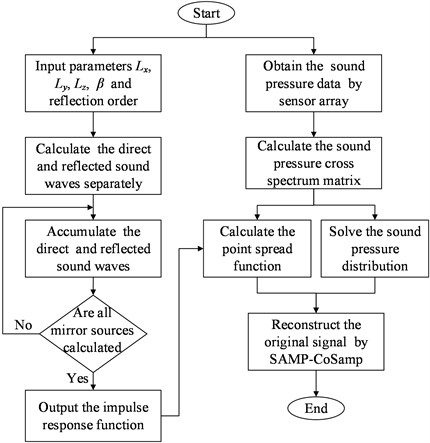In the previous study, a hybrid compressive sensing reconstruction algorithm called SAMP-CoSamp was proposed. For sound source imaging, combines the speed advantage of CoSaMP algorithm and the adaptive sparsity searching ability from the SAMP algorithm. It enhances the acoustic imaging performance in free-field condition since a good balance between running efficiency and reconstruction error is stroke. Details about this part of modeling can be found in the authors’ previous research work . Its naive beamforming mechanism can be described in Table 1.

Table 1Algorithm 1 for SAMP-CoSamp

 1: Input the initial parameters $K$, $L$, and $F$ as 1, $L=s$, and $F=Ф$, respectively2: Calculate the correlation coefficient by $u=\left\{{u}_{j}\left|{u}_{j}=\left|⟨r,{\varphi }_{j}⟩\right|,j=1,2,\dots \rightN\right\}$, extract the index value with respect to the $K$ maximum values and store them in set $F$3: If ${‖{\mathrm{\Phi }}_{F}^{T}y‖}_{2}\le \frac{1-{\delta }_{K}}{\sqrt{1+{\delta }_{K}}}{‖y‖}_{2}$4: $K={K}_{0}+L$5: else6: continue step 27: end8: Calculate the initiate margin by $r=y-{\mathrm{\Phi }}_{F}{\mathrm{\Phi }}_{\mathrm{F}}^{+}y$9: Set the initial parameters as 0, stage = 1, and 110: Calculate the correlation coefficient by $u=\left\{{u}_{j}\left|{u}_{j}=\left|⟨r,{\varphi }_{j}⟩\right|,j=1,2,\dots \rightN\right\}$ and then store the corresponding index value in the index set if${u}_{j}\ge 0.5max\left|u\right|$11: Calculate the correlation coefficient by $u=\left\{{u}_{j}\left|{u}_{j}=\left|⟨r,{\varphi }_{j}⟩\right|,j=1,2,\dots \rightN\right\}$, Then extract the index values with respect to the k maximum values and store them in the new set ${F}_{new}$12: Calculate the estimated signal by ${x}_{new}=argmin{‖y-{\Phi }_{{\stackrel{~}{\Lambda }}_{t}}x‖}_{2}$ and update the margin ${r}_{new}=y-{\mathrm{\Phi }}_{F}x$.13: If ${‖{x}_{new}-x‖}_{2}\le \epsilon$14: stop the iteration 15: else16: skip to step 1817: end 18 : If ${‖{r}_{new}‖}_{2}\ge {‖r‖}_{2}$19: stage = stage + 1, $L=$ stage*s20: else21: $F={F}_{new}$, $r={r}_{new}k=k+1$, Afterwards, return to step 1022: end Parameters description: $y$ → measured sound pressure, $F$ → Final value set corresponding to the index, $x$ → Estimated sound source signal data, $k$ → Iterations number, $s$ → Step size, $u$ → Correlation coefficient, $⟨•,•⟩$ → Operator of vector’s inner product, ${\mathrm{\Phi }}_{j}$→$j$thcolumn measurement matrix

## 3. Simulation analysis

### 3.1. Simulation model building

Simulation is conducted to demonstrate the effectiveness of SC-DAMAS for sound source localisation issues. At first, a reverberation field area with a size of 3 m×4 m×3 m, is constructed in the COMSOL multiphysics simulation environment. Considering the different properties and characteristics of the reverberation field and the free field, we choose the ray acoustics module for modeling and simulation. A monopole source surrounded by air is set as the sound source at (1.5 m, 3 m, 1.5 m), and its power intensity is set to 10e-5 W. In order to better simulate the sound propagation process in the actual environment, the fluid model is set to atmospheric attenuation with the relative humidity of 50 % and the initial ray number of 10e5. When sound waves propagate to each wall surface, reflection and absorption will occur. The amount of reflection and absorption varies with the sound absorption coefficient of each wall surface. The measurement surface of sensor array is set at 1m from the sound source surface, whose array element spacing is 0.1 m, surface size is 1 m×1 m and array elements number is 11×11. The mesh division of the reverberation field and the schematic diagram of the sensor array position are shown in Fig. 2. Besides, it is worth mentioning that During the modeling process, we carefully consider the absorption coefficient of each wall. According to our investigation, the sound absorption coefficient of each wall at different frequencies is also shown in the Table 2.

Fig. 2Simulation model of the sound source in reverberant field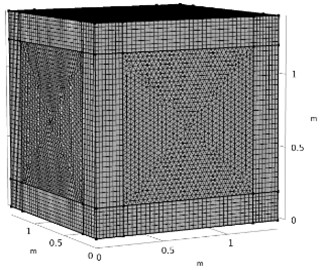a) Mesh division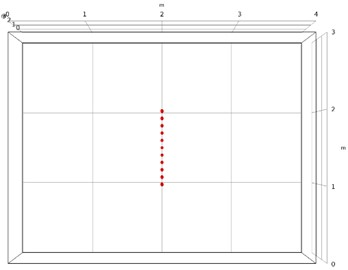b) Schematic diagram of measurement array

Table 2Sound absorption coefficient of each wall of the room

 Frequency (Hz) 125 250 500 1000 2000 4000 Front and left side 0.18 0.06 0.04 0.03 0.02 0.02 Right side and ceiling 0.1 0.05 0.06 0.07 0.09 0.08 Ground 0.01 0.01 0.01 0.01 0.02 0.02 Back side 0.35 0.25 0.18 0.12 0.07 0.04

To further study the applicability of the algorithm in the complex sound field background, the low signal-to-noise ratio factor is added to the reverberation background. More specifically, gaussian white noise with a relative amplitude of 0.01 times, 0.1 times, and 0.3 times is added to the obtained sound pressure data from measurement surface to simulate the noisy environment of SNR = 10 dB, respectively. Taking the collected sound pressure data under the frequency of 2000 Hz and the time of 0.1 s as an instance, Fig. 3 shows the sound pressure distribution in the measurement surface while Gaussian white noise with different relative amplitudes is added.

Fig. 3Sound pressure distribution of different SNR conditions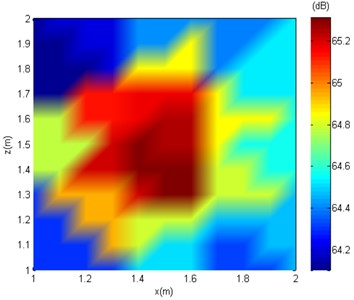a) Noise-free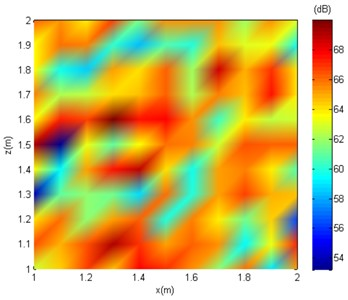b) SNR = 10 dB

It can be seen from Fig. 3, under no-noise conditions, the sound pressure distribution on the measuring surface is relatively concentrated, and the approximate position of the sound source can be judged. After adding noise, the sound pressure distribution on the measuring surface gradually becomes fuzzy or even chaotic with increasing noise, and it is no longer possible to judge the position of the sound source through the sound pressure level distribution on the measuring surface. In the following text, the SC-DAMAS are applied for sound source localisation with the effects of white noise.

### 3.2. Acoustic imaging result

In a practice environment, the target sound source signal could be submerged by noise, especially for low SNR cases, which negatively affects the information extraction and imaging of the target sound source. Therefore, the sound source localisation issues under low SNR conditions is an urgent problem in engineering practice. In the study, the CBF , OMP-DAMAS , and SC-DAMAS are used to process the measured sound field data with SNR = 10 dB under different frequencies. The comparative pressure distributions of different frequency sounds are described in Fig. 4-6.

Fig. 4Comparative imaging results under the frequency of 1000 Hz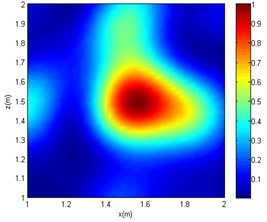a) CBF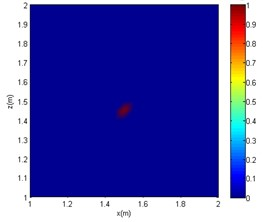b) OMP-DAMAS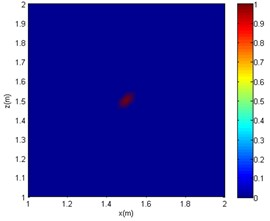c) SC-DAMAS

Fig. 5Comparative imaging results under the frequency of 2000 Hz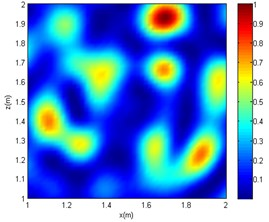a) CBF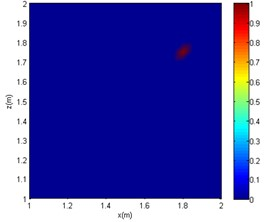b) OMP-DAMAS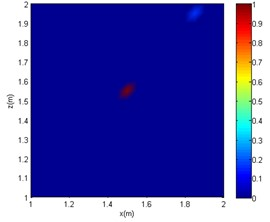c) SC-DAMAS

Fig. 6Comparative imaging results under the frequency of 4000 Hz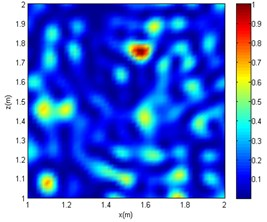a) CBFb) OMP-DAMAS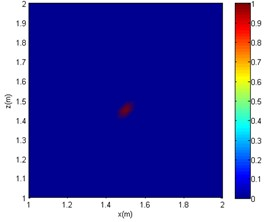c) SC-DAMAS

It can be seen from Figs. 4-6 that in the reverberation field, for CBF, only an approximate range can be obtained at low frequencies. At medium and high frequencies, the acoustic imaging is chaotic, and there is obvious interference with greater intensity, which makes the localisation fail. As for the OMP-DAMAS method, in the reverberation field, the algorithm can maintain a certain degree of accuracy except for the low-frequency conditions. Although the imaging results have no sidelobes in the mid- and high-frequency conditions, they are seriously inconsistent with the actual sound source position. Superior to the CBF and OMP-DAMAS, the SC-DAMAS method has good localisation accuracy in a wide frequency range without sidelobe interference. The specific sound source localisation results by three methods are shown in Table 3.

Table 3Sound source localisation and algorithm efficiency comparison

 Algorithm CBF OMP-DAMAS SC-DAMAS 1000 Hz (1.55 m, 1.5 m) (1.5 m, 1.45 m) (1.5 m, 1.5 m) 2000 Hz (1.7 m, 1.95 m) (1.8 m, 1.75 m) (1.5 m, 1.55 m) 4000 Hz (1.6 m, 1.75 m) (1.15 m, 1.6 m) (1.5 m, 1.45 m)

In summary, under the conditions of the same signal-to-noise ratio and the same sound source frequency, the sound source localisation result of the CBF method is far from the actual sound source position, and a certain intensity of interference sound source would appear. Although there are no interfering sound sources in the imaging results of the OMP-DAMAS method, there is a poor localisation accuracy at mid and high frequencies cases (2000 or 4000 Hz). As the frequency increases, although the OMP-DAMAS imaging results have no obvious sidelobes, there is also the problem of reduced accuracy. The change of frequency has little effect on the SC-DAMAS method, and its imaging results achieve high accuracy, featuring no sidelobe effects, good stability, and reliability.

## 4. Test verification

In order to verify the acoustic imaging effectiveness of the proposed SC-DAMAS for the sound source of low signal-to-noise ratio in reverberant field, acoustic imaging experiments are carried out. An actual ship engine cabin with a size of 17.3 m×8.6 m×2.2 m is selected as the experimental site. As shown in Fig. 7(a), the main noise sources in the cabin are diesel generators and diesel engine. It is worth mentioning that the cabin is confined, and there is other equipment thus environmental noise is unavoidable. Therefore, the cabin forms a reverberation field, and its sound source features low signal-to-noise ratio. During the start of the ship, the main noise sources in the engine room are near the piston cylinder, the intake pipe, and the exhaust pipe. As for the stop phase, the main noise source is near the piston cylinder. In our experiment, both of the ship’s start and stop stages are considered to conduct acoustic imaging experiments. As shown in Fig. 7(b) and (c), the surveyor uses a handheld acoustic imaging test system to collect the sound source data 1.5 meters away.

After the experiment, the measured real-time sound pressure data by the sensor array are exported to the host computer and taken as the input of SC-DAMAS, and the obtained imaging results are shown in Fig. 8. It can be seen that the calculated noise position of the diesel generator during the ship’s start and stop stage is not the same. The main noise source during the start phase appears near the diesel engine piston cylinder, intake pipe, exhaust pipe, etc. As for the case of the stop stage, the main noise comes from the piston cylinder. Similar with the measurement results of diesel generator, the acoustic imaging of diesel engine by SC-DAMAS can locate the noise sources in the two stages. In the start up stage, the main noise source is located around the piston cylinder, intake and exhaust pipe. As for that of stop stage, the main noise is generated by piston cylinder. The Sound source localisation experiment of diesel generator and diesel engine in reverberant field is highly consistent with the actual situation. It is worth mentioning that there is more complex on-site interference than simulation environment, and the positioning accuracy needs to be improved. However, it can be seen in Fig.8 that a sufficiently precise position of noise source has been obtained, which is meaningful since it can give good guidance to engineers and technicians. In summary, the applicability of the reported algorithm for sound source localisation in reverberation is verified.

Fig. 7Sound source localisation experiment in reverberant field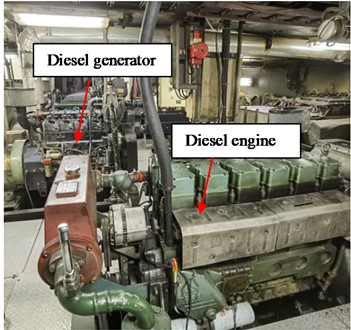a) Ship engine cabin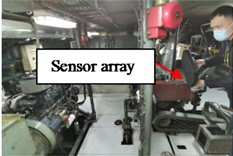b) Diesel generator noise measurement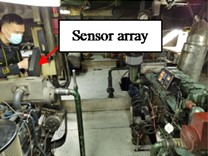c) Diesel engine noise measurement

Fig. 8Experimental result of sound source imaging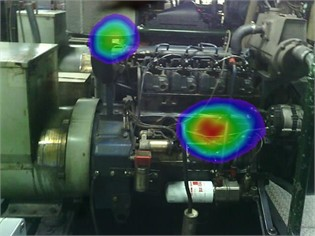a) Star up of diesel generator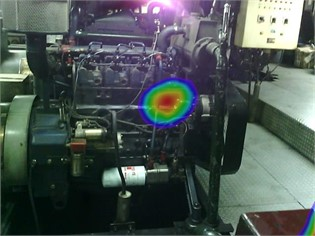b) Stop of diesel generator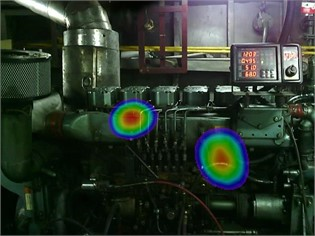c) Star up of diesel engine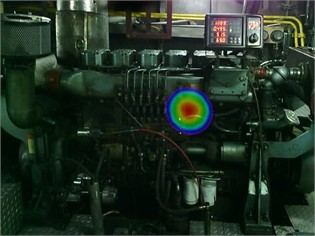d) Stop of diesel engine

## 5. Conclusions

In this paper, an adaptive beamforming algorithm based on impulse response function is proposed in this paper, which can effectively reduce the interference caused by reverberation and low signal-to-noise ratio factors on acoustic imaging. For its adaptive feature, a hybrid compressive sensing reconstruction algorithm called SAMP-CoSaMP is proposed first. This hybrid algorithm not only gets rid of the dependence of the original CoSaMP on the sparsity but also alleviated the problem of inefficiency of the SAMP. Then combined with the DAMAS, the adaptive sound source localisation approach called SC-DAMAS is further put forward. Compared with the CBF and OMP-DAMAS, this method has better imaging accuracy under the background of low signal-to-noise ratio and reverberation, besides it is suitable for a wider frequency range. In the end, taking common marine machinery and equipment as the research object, an acoustic imaging experiment under the background of the relevant reverberation sound field is designed and carried out, which verifies the effectiveness of the algorithm in this paper.

#### Acknowledgements

The authors have not disclosed any funding.